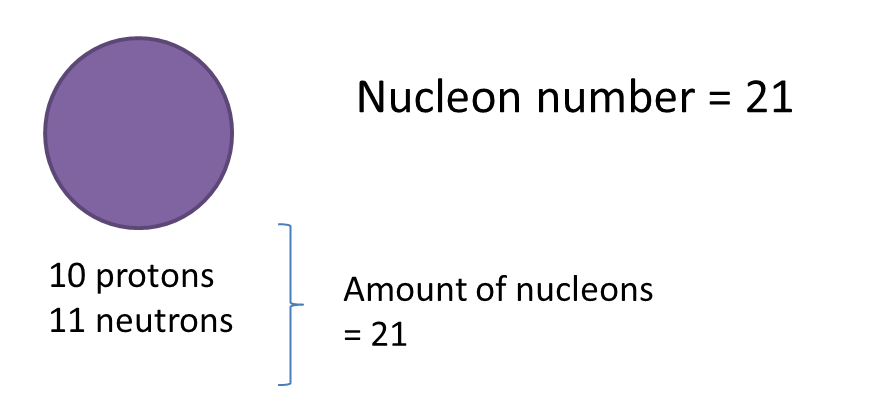4.3 Proton Number and Nucleon Number in Atoms of Elements

4.3 Proton Number and Nucleon Number in Atoms of Elements

4.3.1 Proton Number and Nucleon Number
1. The proton number represents the number of proton found in nucleus of an atom. Sometime, it is also known as the atomic number.

2. Nucleons are the subatomic particles inside the nucleus of an atom,
Nucleon = protons and neutrons

3. Nucleon number represents the number of nucleons in an atom.
Nucleon Number = Number of protons + Number of neutrons

Example:4. In a neutral atom contains an equal number of protons and electrons.

5. Atoms of certain elements can be represented by the following symbol:Number of protons = 8   Number of electrons = 8   Number of neutrons = 16 – 8 = 8

6. Table below shows the proton number and nucleon number for some elements.

 Atom Proton Number Nucleon Number Amount of Proton Amount of electron Amount of Neutron Helium 2 4 2 2 2 Oxygen 8 16 8 8 8 Sodium 11 23 11 11 12 Chlorine 17 35 17 17 18
Relationship between the number of subatomic particles, proton number and nucleon number